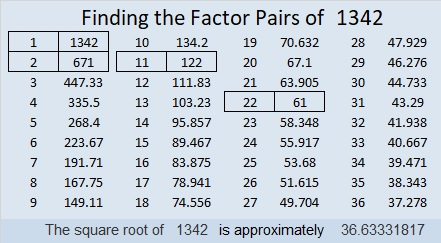# 1342 Level One Puzzles OnlyMy son requested I made some pages using only level 1 puzzles for my granddaughter to do. You can see and use the first page above, but I’ve also compiled ALL the level 1 puzzles from 2018 and put them in one excel file: Level 1’s from 2018

Those puzzles will give beginners lots of practice and help them learn the multiplication table.

Now I’ll tell you a little bit about the number 1342:

• 1342 is a composite number.
• Prime factorization: 1342 = 2 × 11 × 61
• 1342 has no exponents greater than 1 in its prime factorization, so √1342 cannot be simplified.
• The exponents in the prime factorization are 1, 1, and 1. Adding one to each exponent and multiplying we get (1 + 1)(1 + 1)(1 + 1) = 2 × 2 × 2 = 8. Therefore 1342 has exactly 8 factors.
• The factors of 1342 are outlined with their factor pairs in the graphic below.1342 is the hypotenuse of a Pythagorean triple:
242-1320-1342 which is 22 times (11-60-61)

And (11-60-61) times 122 is a triple where 1342 is a leg:
1342-7320-7442. Pretty cool!

The last prime number was 1327 which was 15 numbers ago. The next prime number won’t be until 1361 which is 19 numbers away. Wow! That means that 1342 is 15 numbers away from the CLOSEST prime number, a new record. Thank you OEIS.org for alerting me to that fact.

This site uses Akismet to reduce spam. Learn how your comment data is processed.